2022-05-24
###### Common mistakes to avoid as a Machine Learning Engineer
2022-05-26

Nowadays, there is an infinite number of applications that someone can do with Deep Learning. However, in order to understand the plethora of design choices such as skip connections that you see in so many works, it is critical to understand a little bit of the mechanisms of backpropagation.

If you were trying to train a neural network back in 2014, you would definitely observe the so-called vanishing gradient problem. In simple terms: you are behind the screen checking the training process of your network and all you see is that the training loss stops decreasing but it is still far away from the desired value. You check all your code lines to see if something was wrong all night and you find no clue. Not the best experience in the world, believe me!

## The problem

The beauty of deep neural networks is that they can learn complex functions more efficiently than their shallow counterparts. While training deep neural nets, the performance of the model drops down with the increase in depth of the architecture. This is known as the degradation problem. But, what could be the reasons for the saturation inaccuracy with the increase in network depth? Let us try to understand the reasons behind the degradation problem.

One of the possible reasons could be overfitting. The model tends to overfit with the increase in depth but that’s not the case here.  As you can infer from the below figure, the deeper network with 56 layers has more training error than the shallow one with 20 layers. The deeper model doesn’t perform as well as the shallow one.  Clearly, overfitting is not the problem here.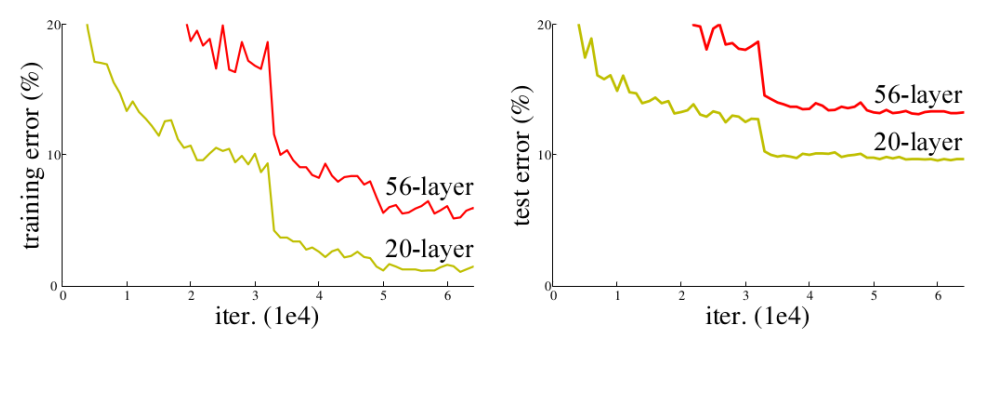Train and test error for 20-layer and 56-layer NN

Another possible reason can be vanishing gradient and/or exploding gradient problems. However, the authors of ResNet (He et al.) argued that the use of Batch Normalization and proper initialization of weights through normalization ensures that the gradients have healthy norms. But, what went wrong here? Let’s understand this by construction.

Consider a shallow neural network that was trained on a dataset. Also, consider a deeper one in which the initial layers have the same weight matrices as the shallow network (the blue colored layers in the below diagram) with added some extra layers (green colored layers). We set the weight matrices of the added layers as identity matrices (identity mappings).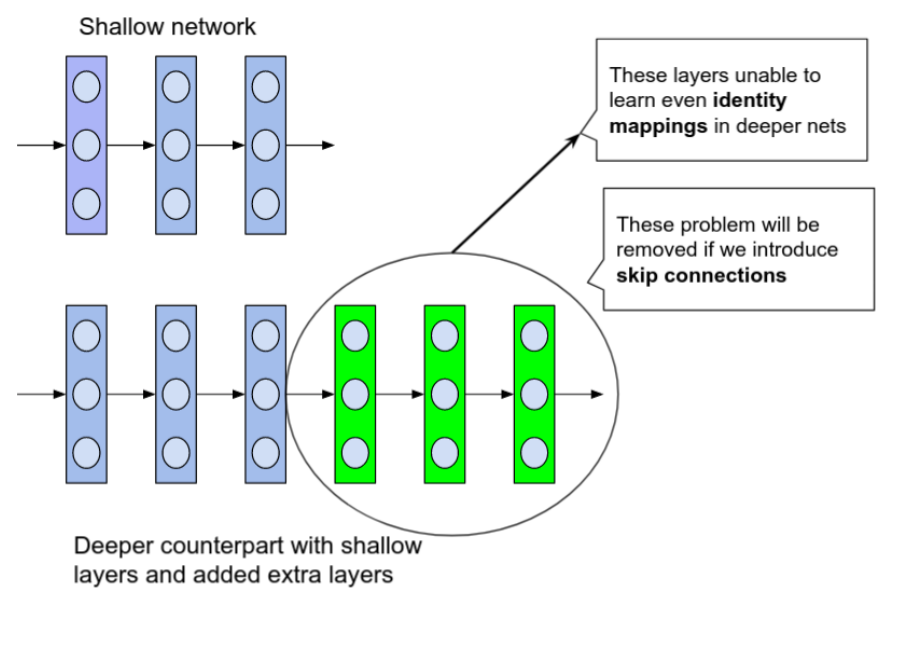Diagram explaining the construction

From this construction, the deeper network should not produce any higher training error than its shallow counterpart because we are actually using the shallow model’s weight in the deeper network with added identity layers. But experiments prove that the deeper network produces high training error compared to the shallow one. This states the inability of deeper layers to learn even identity mappings.

The degradation of training accuracy indicates that not all systems are similarly easy to optimize.

One of the primary reasons is due to random initialization of weights with a mean around zero, L1, and L2 regularization.  As a result, the weights in the model would always be around zero and thus the deeper layers can’t learn identity mappings as well.

## The update rule and the vanishing gradient problem

So, let’s remind our self the update rule of gradient descent without momentum, given L to be the loss function and \lambdaλ the learning rate:where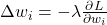What is basically happening is that you try to update the parameters by changing them with a small amount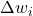that was calculated based on the gradient, for instance, let’s suppose that for an early layer the average gradient 1e-15 (). Given a learning rate of 1e-4 ( λ in the equation), you basically change the layer parameters by the product of the referenced quantities, which is 1e-19 (). As a result, you don’t actually observe any change in the model while training your network. This is how you can observe the vanishing gradient problem.

Looking a little bit at the theory, one can easily grasp the vanishing gradient problem from the backpropagation algorithm. We will briefly inspect the backpropagation algorithm from the prism of the chain rule, starting from basic calculus to gain an insight into skip connections.

In short, backpropagation is the “optimization-magic” behind deep learning architectures. Given that a deep network consists of a finite number of parameters that we want to learn, our goal is to iteratively optimize these parameters with respect to the loss function L.

As you have seen, each architecture has some input (i.e. an image) and produces an output (prediction). The loss function is heavily based on the task we want to solve. For now, what you need to know is the loss function is a quantitative measure of the distance between two tensors, that can represent an image label, a bounding box in an image, a translated text in another language, etc. You usually need some kind of supervision to compare the network’s prediction with the desired outcome (ground truth). Keep in mind that backpropagation belongs in the supervised machine learning category.

So, the beautiful idea of backpropagation is to gradually minimize this loss by updating the parameters of the network. But how can you propagate the scalar measured loss inside the network? That’s exactly where backpropagation comes into play.

## Backpropagation and partial derivatives

In simple terms, backpropagation is about understanding how changing the weights (parameters) in a network changes the loss function by computing the partial derivatives. For the latter, we use the simple idea of the chain rule, to minimize the distance in the desired predictions. In other words, backpropagation is all about calculating the gradient of the loss function while considering the different weights within that neural network, which is nothing more than calculating the partial derivatives of the loss function with respect to model parameters. By repeating this step many times, we will continually minimize the loss function until it stops reducing, or some other predefined termination criteria are met.

### Chain rule

The chain rule basically describes the gradient (change) of a loss function i.e. z with respect to some neural network parameter, let’s say x and y which are functions of a previous layer parameter t. Let f, g, and h be different layers on the network that perform a non-linear operation in the input vector.Now, suppose that you are learning calculus and you want to express the gradient of z with respect to the input. This is what you learn in multi-variable calculus:Interestingly, the famous algorithm does exactly the same operation but in the opposite way: it starts from the output z and calculates the partial derivatives of each parameter, expressing it only based on the gradients of the later layers.

It’s really worth noticing that all these values are often less than 1, independent of the sign. In order to propagate the gradient to the earlier layers, backpropagation uses the multiplication of the partial derivatives (as in the chain rule). In general, multiplication with an absolute value less than 1 is nice because it provides some sense of training stability, although there is not a strict mathematic theorem about that. However, one can observe that for every layer that we go backward in the network the gradient of the network gets smaller and smaller.

## Skip connections for the win

Skip Connections (or Shortcut Connections) as the name suggests skips some of the layers in the neural network and feeds the output of one layer as the input to the next layers.

Skip Connections were introduced to solve different problems in different architectures. In the case of ResNets, skip connections solved the degradation problem that we addressed earlier whereas, in the case of DenseNets, it ensured feature reusability. We’ll discuss them in detail in the following sections.

Skip connections were introduced in literature even before residual networks. For example, Highway Networks (Srivastava et al.) had skip connections with gates that controlled and learned the flow of information to deeper layers. This concept is similar to the gating mechanism in LSTM. Although ResNets is actually a special case of Highway networks, the performance isn’t up to the mark compared to ResNets. This suggests that it’s better to keep the gradient highways clear than to go for any gates – simplicity wins here!

Neural networks can learn any functions of arbitrary complexity, which could be high-dimensional and non-convex. Visualizations have the potential to help us answer several important questions about why neural networks work. And there is actually some nice work done by Li et al. which enables us to visualize the complex loss surfaces. The results from the networks with skip connections are even more surprising! Take a look at them.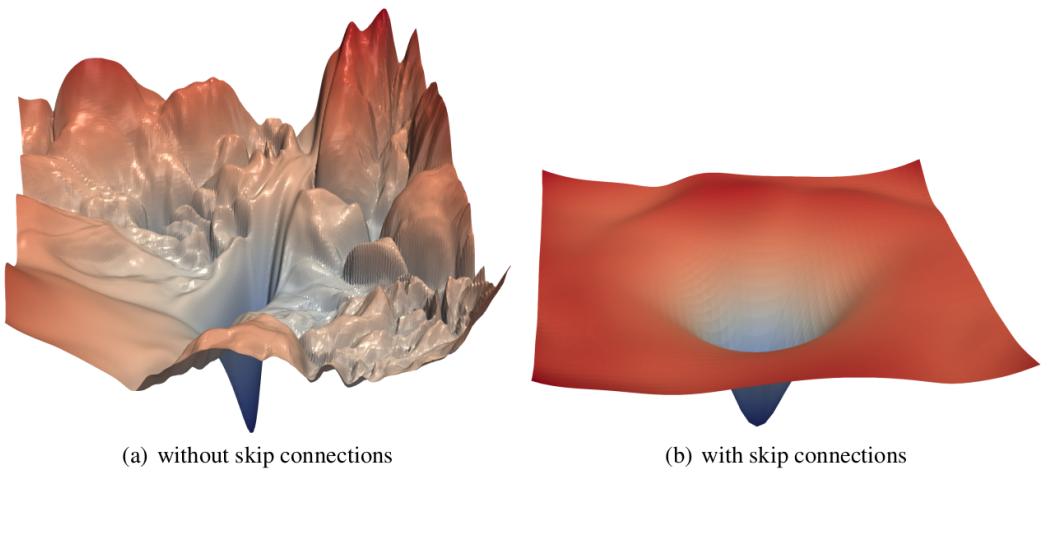The loss surfaces of ResNet-56 with and without skip connections

As you can see here, the loss surface of the neural network with skip connections is smoother and thus leading to faster convergence than the network without any skip connections.

At present, skip connection is a standard module in many convolutional architectures. By using a skip connection, we provide an alternative path for the gradient (with backpropagation). It is experimentally validated that these additional paths are often beneficial for model convergence. Skip connections in deep architectures, as the name suggests, skip some layer in the neural network and feed the output of one layer as the input to the next layers (instead of only the next one).

As previously explained, using the chain rule, we must keep multiplying terms with the error gradient as we go backward. However, in the long chain of multiplication, if we multiply many things together that are less than one, then the resulting gradient will be very small. Thus, the gradient becomes very small as we approach the earlier layers in a deep architecture. In some cases, the gradient becomes zero, meaning that we do not update the early layers at all.

In general, there are two fundamental ways that one could use skip connections through different non-sequential layers:

a) addition as in residual architectures,

b) concatenation as in densely connected architectures.

We will first describe addition which is commonly referred to as residual skip connections.

## ResNet: skip connections via addition

The core idea is to backpropagate through the identity function, by just using vector addition. Then the gradient would simply be multiplied by one and its value will be maintained in the earlier layers. This is the main idea behind Residual Networks (ResNets): they stack these skip residual blocks together. We use an identity function to preserve the gradient.

Mathematically, we can represent the residual block, and calculate its partial derivative (gradient), given the loss function like this: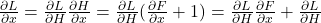Apart from the vanishing gradients, there is another reason that we commonly use them. For a plethora of tasks (such as semantic segmentation, optical flow estimation, etc.) there is some information that was captured in the initial layers and we would like to allow the later layers to also learn from them. It has been observed that in earlier layers the learned features correspond to lower semantic information that is extracted from the input. If we had not used the skip connection that information would have turned too abstract.

## DenseNet: skip connections via concatenation

As stated, for many dense prediction problems, there is low-level information shared between the input and output, and it would be desirable to pass this information directly across the net. The alternative way that you can achieve skip connections is by concatenation of previous feature maps. The most famous deep learning architecture is DenseNet.  Below you can see an example of feature reusability by concatenation with 5 convolutional layers:

This architecture heavily uses feature concatenation so as to ensure maximum information flow between layers in the network. This is achieved by connecting via concatenation all layers directly with each other, as opposed to ResNets. Practically, what you basically do is to concatenate the feature channel dimension. This leads to

a) an enormous amount of feature channels on the last layers of the network,

b) to more compact models, and

c) extreme feature reusability.

## Short and Long skip connections in Deep Learning

In more practical terms, you have to be careful when introducing additive skip connections in your deep learning model. The dimensionality has to be the same in addition and also in concatenation apart from the chosen channel dimension. That is the reason why you see that additive skip connections are used in two kinds of setups:

a) short skip connections

b) long skip connections.

Short skip connections are used along with consecutive convolutional layers that do not change the input dimension (see Res-Net), while long skip connections usually exist in encoder-decoder architectures. It is known that the global information (shape of the image and other statistics) resolves whatwhile local information resolves where (small details in an image patch).

Long skip connections often exist in architectures that are symmetrical, where the spatial dimensionality is reduced in the encoder part and is gradually increased in the decoder part as illustrated below. In the decoder part, one can increase the dimensionality of a feature map via transpose convolutional layers. The transposed convolution operation forms the same connectivity as the normal convolution but in the backward direction.

## U-Nets: long skip connections

Mathematically, if we express convolution as matrix multiplication, then transpose convolution is the reverse order multiplication (BxA instead of AxB). The aforementioned architecture of the encoder-decoder scheme along with long skip connections is often referred to as U-shape (Unet). It is utilized for tasks that the prediction has the same spatial dimension as the input such as image segmentation, optical flow estimation, video prediction, etc.

Long skip connections can be formed in a symmetrical manner, as shown in the diagram below:

By introducing skip connections in the encoder-decoded architecture, fine-grained details can be recovered in the prediction. Even though there is no theoretical justification, symmetrical long skip connections work incredibly effectively in dense prediction tasks (medical image segmentation).

Okay! Enough of theory, let’s implement a block of the discussed architectures and how to load and use them in PyTorch!

## Implementation of Skip Connections

In this section, we will build ResNets and DesNets using Skip Connections from the scratch. Are you excited? Let’s go!

### ResNet – A residual block

First, we will implement a residual block using skip connections. PyTorch is preferred because of its super cool feature – object-oriented structure.

# import required libraries
import torch
from torch import nn
import torch.nn.functional as F
import torchvision

# basic resdidual block of ResNet
# This is generic in the sense, it could be used for downsampling of features.
class ResidualBlock(nn.Module):
def __init__(self, in_channels, out_channels, stride=[1, 1], downsample=None):
"""
A basic residual block of ResNet
Parameters
----------
in_channels: Number of channels that the input have
out_channels: Number of channels that the output have
stride: strides in convolutional layers
downsample: A callable to be applied before addition of residual mapping
"""
super(ResidualBlock, self).__init__()

self.conv1 = nn.Conv2d(
in_channels, out_channels, kernel_size=3, stride=stride,
)

self.conv2 = nn.Conv2d(
out_channels, out_channels, kernel_size=3, stride=stride,
)

self.bn = nn.BatchNorm2d(out_channels)
self.downsample = downsample

def forward(self, x):
residual = x
# applying a downsample function before adding it to the output
if(self.downsample is not None):
residual = downsample(residual)

out = F.relu(self.bn(self.conv1(x)))

out = self.bn(self.conv2(out))
# note that adding residual before activation
out = out + residual
out = F.relu(out)
return out


As we have a Residual block in our hand, we can build a ResNet model of arbitrary depth! Let’s quickly build the first five layers of ResNet-34 to get an idea of how to connect the residual blocks.

# downsample using 1 * 1 convolution
downsample = nn.Sequential(
nn.Conv2d(64, 128, kernel_size=1, stride=2, bias=False),
nn.BatchNorm2d(128)
)
# First five layers of ResNet34
resnet_blocks = nn.Sequential(
nn.Conv2d(3, 64, kernel_size=7, stride=2, padding=3, bias=False),
nn.MaxPool2d(kernel_size=2, stride=2),
ResidualBlock(64, 64),
ResidualBlock(64, 64),
ResidualBlock(64, 128, stride=[2, 1], downsample=downsample)
)

# checking the shape
inputs = torch.rand(1, 3, 100, 100) # single 100 * 100 color image
outputs = resnet_blocks(inputs)
print(outputs.shape)    # shape would be (1, 128, 13, 13)


PyTorch provides us an easy way to load ResNet models with pretrained weights trained on the ImageNet dataset.

# one could also use pretrained weights of ResNet trained on ImageNet
resnet34 = torchvision.models.resnet34(pretrained=True)


### DenseNet – A dense block

Implementing the complete DenseNet would be a little bit complex. Let’s grab it step by step.

1. Implement a DenseNet layer
2. Build a dense block
3. Connect multiple dense blocks to obtain a DenseNet model
class Dense_Layer(nn.Module):
def __init__(self, in_channels, growthrate, bn_size):
super(Dense_Layer, self).__init__()

self.bn1 = nn.BatchNorm2d(in_channels)
self.conv1 = nn.Conv2d(
in_channels, bn_size * growthrate, kernel_size=1, bias=False
)
self.bn2 = nn.BatchNorm2d(bn_size * growthrate)
self.conv2 = nn.Conv2d(
bn_size * growthrate, growthrate, kernel_size=3, padding=1, bias=False
)

def forward(self, prev_features):
out1 = torch.cat(prev_features, dim=1)
out1 = self.conv1(F.relu(self.bn1(out1)))
out2 = self.conv2(F.relu(self.bn2(out1)))
return out2


Next, we’ll implement a dense block that consists of an arbitrary number of DenseNet layers.

class Dense_Block(nn.ModuleDict):
def __init__(self, n_layers, in_channels, growthrate, bn_size):
"""
A Dense block consists of n_layers of Dense_Layer
Parameters
----------
n_layers: Number of dense layers to be stacked
in_channels: Number of input channels for first layer in the block
growthrate: Growth rate (k) as mentioned in DenseNet paper
bn_size: Multiplicative factor for # of bottleneck layers
"""
super(Dense_Block, self).__init__()

layers = dict()
for i in range(n_layers):
layer = Dense_Layer(in_channels + i * growthrate, growthrate, bn_size)
layers['dense{}'.format(i)] = layer

self.block = nn.ModuleDict(layers)

def forward(self, features):
if(isinstance(features, torch.Tensor)):
features = [features]

for _, layer in self.block.items():
new_features = layer(features)
features.append(new_features)



From the dense block, let’s build DenseNet. Here, I’ve omitted the transition layers of DenseNet architecture (which acts as downsampling) for simplicity.

# a block consists of initial conv layers followed by 6 dense layers
dense_block = nn.Sequential(
nn.Conv2d(3, 64, kernel_size=7, padding=3, stride=2, bias=False),
nn.BatchNorm2d(64),
nn.MaxPool2d(3, 2),
Dense_Block(6, 64, growthrate=32, bn_size=4),
)

inputs = torch.rand(1, 3, 100, 100)
outputs = dense_block(inputs)
print(outputs.shape)    # shape would be (1, 256, 24, 24)

# one could also use pretrained weights of DenseNet trained on ImageNet
densenet121 = torchvision.models.densenet121(pretrained=True)


## Conclusion

To sum up, the motivation behind skip connections is that they have an uninterrupted gradient flow from the first layer to the last layer, which tackles the vanishing gradient problem. Concatenative skip connections enable an alternative way to ensure feature reusability of the same dimensionality from the earlier layers and are widely used.

On the other hand, long skip connections are used to pass features from the encoder path to the decoder path in order to recover spatial information lost during downsampling. Short skip connections appear to stabilize gradient updates in deep architectures. Finally, skip connections enable feature reusability and stabilize training and convergence.

As a final note, encouraging further reading, it has been experimentally validated [Li et al 2018] that the loss landscape changes significantly when introducing skip connections.

Resources:

https://theaisummer.com/skip-connections/

https://www.analyticsvidhya.com/blog/2021/08/all-you-need-to-know-about-skip-connections/##### Amir Masoud Sefidian
Machine Learning Engineer